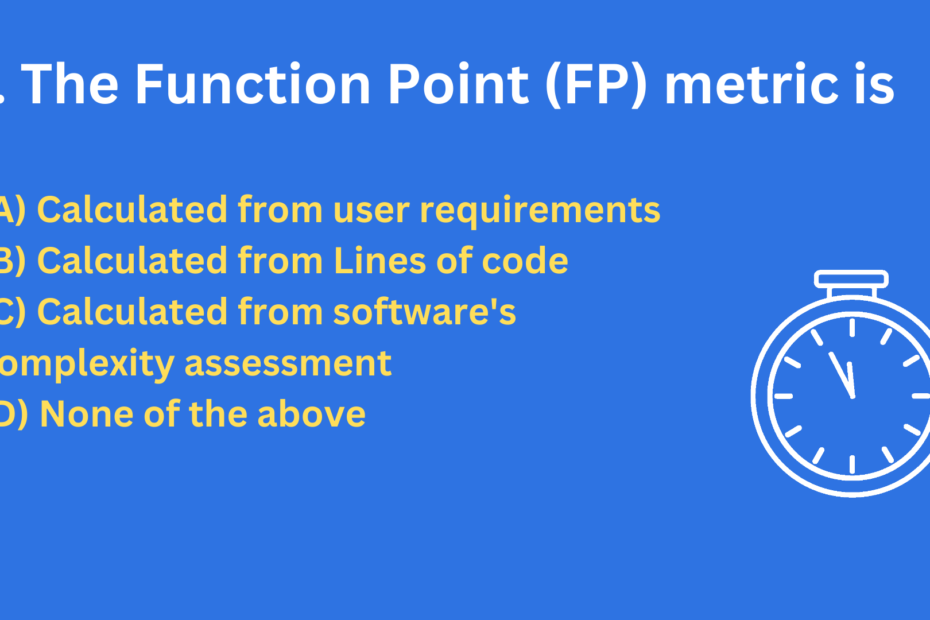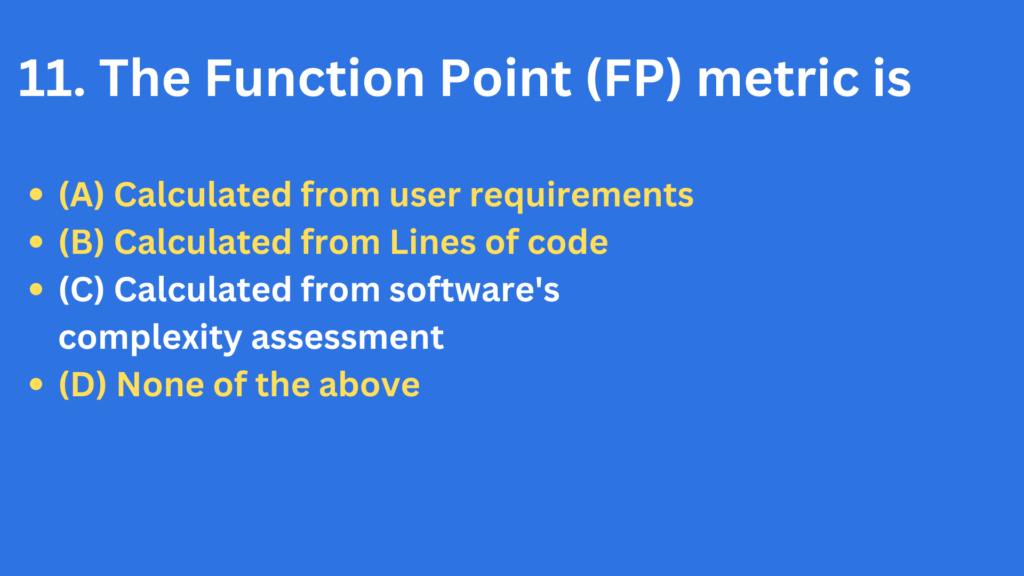# The Function Point (FP) metric is

• CS PYQ## The Function Point (FP) metric is

• (A) Calculated from user requirements
• (B) Calculated from Lines of code
• (C) Calculated from the software’s complexity assessment
• (D) None of the above

## Function Point Analysis (FPA)

Function Point Analysis (FPA) is a method used to estimate the complexity and size of software systems and applications. It was developed in the 1970s as a way to measure the functionality provided by an information system, independent of the technology used to implement it.

Function points are a measure of the functionality provided by an information system, expressed in terms of the number of user functions it supports. Each function point represents the equivalent of one user function, such as a data entry screen or a report.

To perform FPA, you first identify the functions provided by the system, and then assign a value to each function based on its complexity. The sum of these values is the total number of function points for the system. This number can then be used to estimate the time and resources required to develop or maintain the system, as well as its potential value to the organization.

Function points can be used to compare the functionality provided by different systems, or to track changes in the functionality of a single system over time. They are often used in conjunction with other software metrics, such as lines of code or defect density, to provide a more complete picture of the software development process.

The Function Point (FP) metric is Calculated from software’s complexity assessment